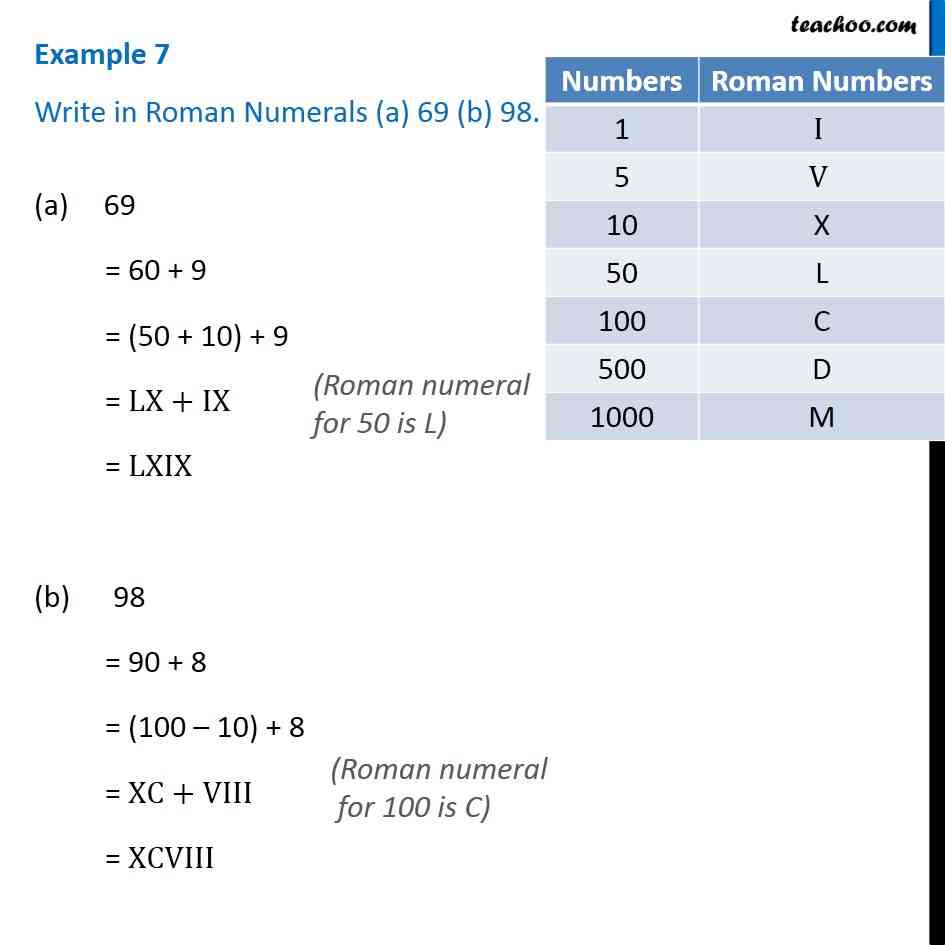Examples

Chapter 1 Class 6 Knowing our Numbers
Serial order wiseLearn in your speed, with individual attention - Teachoo Maths 1-on-1 Class

### Transcript

Question 3 Write in Roman Numerals (a) 69 (b) 98. 69 = 60 + 9 = (50 + 10) + 9 = LX+IX = LXIX (b) 98 = 90 + 8 = (100 10) + 8 = XC+VIII = XCVIII (Roman numeral for 50 is L) (Roman numeral for 100 is C) (Roman numeral for 100 is C) (Roman numeral for 100 is C)